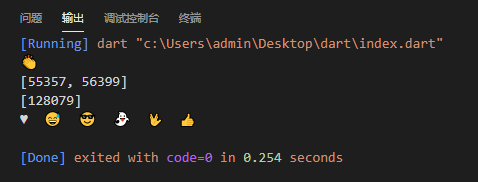Dart语言基础，Dart 基础顺心130 0 0
1. Dart 的main方法有两种声明方式
// 第一种声明方式
main(){
print("hello world");  //打印
};
// 第二种声明方式.如果有学过typeScript或其它语言的同学都明白下面代码的意思，这个方法是有没返回值的。
void main (){
print("hello world");
}; 
1. 注释
// 这个是一个单行注释
/*

*/
/// 这也是注释 
1. 变量的声明 、命名规则、数组类型

Dart是一个强大的脚本类语言，可以不预先定义类型，自动会类型推断
Dart 中定义变量也可以使用var关键字来声明
Dart 变量命名规则：
1.变量名称必须由数字、字母、下划线和美元符(\$)组成
2.注意：标识符开头不能是数字
3.标识符不能是保留字或关键字
4.变量的名字是区分大小写如：age和Age是不同的变量，在实际的项目中不建议这样使用
5.变量名一定要见名思意：变量名称建议用名词，方法名称建议用动词

Dart中常用数据类型：

String (字符串);

Int (整数)
double (浮点数)
List （集合/数组）在Dart中，数组是列表对象，所以大多数同学都是称它为列表
Booleans(布尔)
Maps(字典/跟js的object相似) 通常来说,Map 是一个键值对关的对象，键和值可以任何类型的对象，每个键只

main() {
var str = "我是字符串1"; // 自动会类型推断
String as1 = "我是字符串as1"; // 定义g一个字符串
int numbers = 10; // 定义g一个整数
double floating=10.1; // 定义g一个浮点数
bool flag= true;   // 值 true / false
var l1=['aaa','bbbb','ccccc']; // 第一种定义List的方式
var l2=new List(); // 第二种定义List的方式
var l3 = new List<String>(); //  指定类型声明
print(l1); // 访问l1的第0个值

var person={ // 第一种定义Map的方式
"name":"qiyingcong",
"age":21
};

var p = new Map(); // 第二种定义Map的方式
p["name"] = "qi"; // 赋值
print(person["name"]); // 访问name

// is 关键词判断数据类型
print(str is String);

int subTot = str; // 这样会报错，因为string类型不直接赋值给int类型

print(str);
print(as1);
print(numbers);
print(floating);
print(flag);
} 

List里面常用的属性和方法：

length 长度
reversed 翻转
isEmpty 是否为空
isNotEmpty 是否不为空

indexOf 查找 传入具体值
remove 删除 传入具体值
removeAt 删除 传入索引值
fillRange 修改
insert(indexer,value) 指定位置插入
inserAll(index,list) 指定位置插入List
toList() 其它类型转换成List
join() List转换成字符串
split() 字符串转化为List
forEach
map
where
any
every

Runes 、Symbols

Runes 是UTF-32编码的字符串，它可以通过文字转换成符号表情或代表特定的文字。

main() {
var clapping = '\u{1f44f}';
print(clapping);
print(clapping.codeUnits);
print(clapping.runes.toList());

Runes input = new Runes('\u2665  \u{1f605}  \u{1f60e}  \u{1f47b}  \u{1f596}  \u{1f44d}');
print(new String.fromCharCodes(input));
}image.png

Symbol对象表示在Dart程序中声明的运算符或标识符。您可能永远不需要使用符号，但它们对于按名称引用标识符的API非常有用，因为缩小会更改标识符名称而不会更改标识符符号。要获取标识符的符号，请使用符号文字，它只是＃后跟标识符：

#radix
#bar 

3.常量的声明 const 和 final

const 值必须在编译时知道,初始化后无法更改
final 值必须在运行时知道,初始化后无法更改

final 可以开始不赋值 只能赋一次；而final不仅有const 的编译时常量的特性，最重要的它是运行时常量，并且final是惰性初始化，在运行时第一次使用前才初始化。

// 比如： const time = '2020-01-01'。
const time = DateTime.now() // 会报错 因为我们无法将运行时值分配给 const 变量
final time = DateTime.now() // 成功 

4.运算符、条件判断、类型转换

int a =14;
int b = 5;

print(a+b); // 加
print(a-b); // 减
print(a*b); // 乘
print(a/b); // 除
print(a%b); // 除余
print(a~/b); // 取整

print(a==b); // 判断是否相等
print(a!=b); // 判断是否不等
print(a>b); // 判断是否大于
print(a<b); // 判断是否小于
print(a>=b); // 判断是否大于等于
print(a<=b); // 判断是否小于等于

逻辑运算符：! 取反   && 并且：全部为true的话值为true 否则为false   || 或者：全部为false则为false

基础赋值运算符： =  ??=
复合赋值运算符：+=    -=    *=     /=    %=     ~/=       ++    --

var a;
var b = a?? 10;  // 两个问号的意思是a为空时会使用10来赋值
print(b); // b的值是10

String price= "123.1";
try {
var myNum = double.parse(price);  // 把str转换成double
} catch(err) {
// 如果转换错误就在这里处理
}
var myNum = 12;
var str = myNum.toString(); // 把int 类型转String

var string1 = '';
// isEmpty:判断字符串是否为空

if (string1.isEmpty) {
print('string1是空');
} else {
print('string1不为空');
}

var sex = "男";
switch (sex) {
case "女":
print("性别女");
break;
case "男":
print("性别男");
break;
default:
print("传入参数有误");
break;
} 

5.循环语句:for、while 、do..while 、break

for(int i =0;i<=10;i++){ //打印0到10
print(i);
}

int i =1;
whild(i<=10){
print(i);
i++;
}

int s = 1;
var sum = 0;
do{
sum+=i;
i++
}while(i<=100);
print(sum);